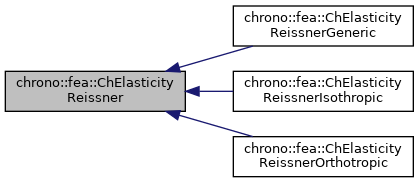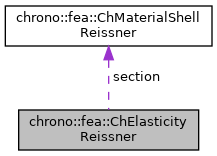chrono::fea::ChElasticityReissner Class Referenceabstract

## Description

Base interface for elasticity of 6-field Reissner-Mindlin shells (kinematically-exact shell theory as in Witkowski et al.) to be used in a ChMaterialShellReissner.

Children classes must implement the ComputeStress function to get {n_u,n_v,m_u,m_v}=f({e_u,e_v,k_u,k_v}) Inherited materials do not define any thickness, which should be a property of the element or its layer(s) using this material.

#include <ChMaterialShellReissner.h>

Inheritance diagram for chrono::fea::ChElasticityReissner:[legend]
Collaboration diagram for chrono::fea::ChElasticityReissner:[legend]

## Public Member Functions

virtual void ComputeStress (ChVector<> &n_u, ChVector<> &n_v, ChVector<> &m_u, ChVector<> &m_v, const ChVector<> &eps_u, const ChVector<> &eps_v, const ChVector<> &kur_u, const ChVector<> &kur_v, const double z_inf, const double z_sup, const double angle)=0
Compute the generalized force and torque, given actual deformation and curvature. More...

virtual void ComputeStiffnessMatrix (ChMatrixRef mC, const ChVector<> &eps_u, const ChVector<> &eps_v, const ChVector<> &kur_u, const ChVector<> &kur_v, const double z_inf, const double z_sup, const double angle)
Compute the 12x12 stiffness matrix [Km] , that is [ds/de], the tangent of the constitutive relation stresses/strains. More...

## Public Attributes

ChMaterialShellReissnersection

## ◆ ComputeStiffnessMatrix()

 void chrono::fea::ChElasticityReissner::ComputeStiffnessMatrix ( ChMatrixRef mC, const ChVector<> & eps_u, const ChVector<> & eps_v, const ChVector<> & kur_u, const ChVector<> & kur_v, const double z_inf, const double z_sup, const double angle )
virtual

Compute the 12x12 stiffness matrix [Km] , that is [ds/de], the tangent of the constitutive relation stresses/strains.

By default, it is computed by backward differentiation from the ComputeStress() function, but inherited classes should better provide an analytical form, if possible.

Parameters
 mC tangent matrix eps_u strains along u direction eps_v strains along v direction kur_u curvature along u direction kur_v curvature along v direction z_inf layer lower z value (along thickness coord) z_sup layer upper z value (along thickness coord) angle layer angle respect to x (if needed)

## ◆ ComputeStress()

 virtual void chrono::fea::ChElasticityReissner::ComputeStress ( ChVector<> & n_u, ChVector<> & n_v, ChVector<> & m_u, ChVector<> & m_v, const ChVector<> & eps_u, const ChVector<> & eps_v, const ChVector<> & kur_u, const ChVector<> & kur_v, const double z_inf, const double z_sup, const double angle )
pure virtual

Compute the generalized force and torque, given actual deformation and curvature.

This MUST be implemented by subclasses.

Parameters
 n_u forces along u direction (per unit length) n_v forces along v direction (per unit length) m_u torques along u direction (per unit length) m_v torques along v direction (per unit length) eps_u strains along u direction eps_v strains along v direction kur_u curvature along u direction kur_v curvature along v direction z_inf layer lower z value (along thickness coord) z_sup layer upper z value (along thickness coord) angle layer angle respect to x (if needed)

The documentation for this class was generated from the following files:
• /builds/uwsbel/chrono/src/chrono/fea/ChMaterialShellReissner.h
• /builds/uwsbel/chrono/src/chrono/fea/ChMaterialShellReissner.cpp